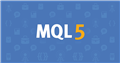# How to extract values form for function and create an array

Hello all

I want to create an array from  9 values and then measure mean of the array. I used the Operator 'for' but I do not know how to extract values from it. want to do is to extract these trmax values from the loop and use it out of the loop. Any idea how to do so? Here is my code:

```for(int i=0;i<=9;i++)
{
double one=High[i] - Low[i];
double two=fabs(High[i] - Close[i+1]);
double three=fabs(Low[i] - Close[i+1]);
ArrayResize(tr,3);
ArrayFill(tr,0,1,(NormalizeDouble(one,Digits)));
ArrayFill(tr,1,1,(NormalizeDouble(two,Digits)));
ArrayFill(tr,2,1,(NormalizeDouble(three,Digits)));
double trmax=tr[ArrayMaximum(tr)];

}```

Hello all

I want to create an array from  9 values and then measure mean of the array. I used the Operator 'for' but I do not know how to extract values from it. want to do is to extract these trmax values from the loop and use it out of the loop. Any idea how to do so? Here is my code:

Create you variables outside the loop. If you create them inside, they get destroyed at the end of each iteration.

Hello,

Setting them in the void OnInit() {} function would be the best option.

So you need to save the values outside of the function it runs in. Pretend you are taking 9 steps and for each step you write down the distance.

If you save the values inside the function, it's like writing down the same number for each step you take. You need to use that step to write down that value inside it.

So let's say you use the onTimer function after you've set the size of the array (using arrayResize).

Let's call your array arrThree; So the data type would be double[], because it is a decimal (which is what a double is) and the [] means it is an array holding multiple values.

Then to save the first value in the array, you would use arrThree.

To save the second value, you would use arrThree;

And then you do this up to arrThree.

But this is why you have a loop. You don't need to insert a decimal at step 0 or 9. You can just use i instead.Documentation on MQL5: Constants, Enumerations and Structures / Named Constants / Predefined Macro Substitutions
• www.mql5.com
//| Expert initialization function                                   | //| Expert deinitialization function                                 | //| Expert tick function                                             | //| test1                                                            |...

little.trader: Setting them in the void OnInit() {} function would be the best option.

Don't try to use any price or server related functions in OnInit (or on load), as there may be no connection/chart yet:

1. Terminal starts.
2. Indicators/EAs are loaded. Static and globally declared variables are initialized. (Do not depend on a specific order.)
3. OnInit is called.
4. For indicators OnCalculate is called with any existing history.
5. Human may have to enter password, connection to server begins.
6. New history is received, OnCalculate called again.
7. New tick is received, OnCalculate/OnTick is called. Now TickValue, TimeCurrent, account information and prices are valid.